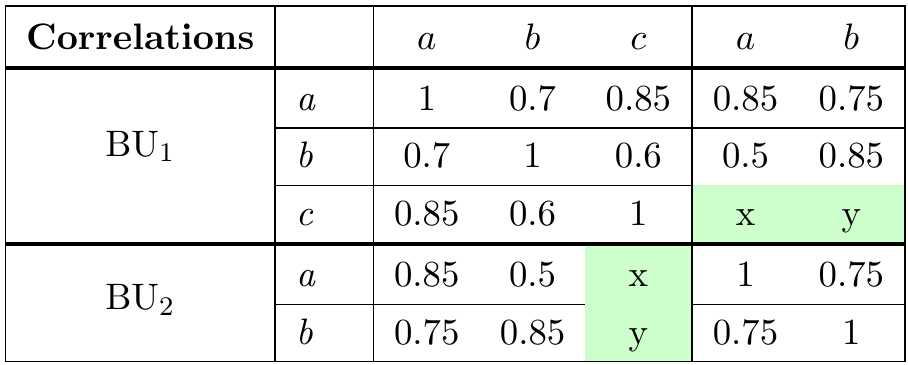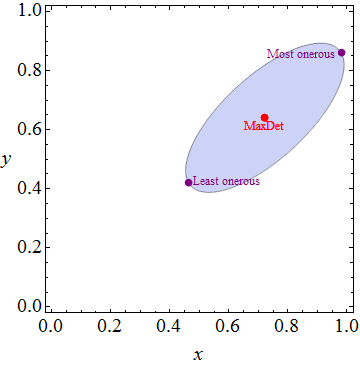# Completing Correlation Matrices

Dan Georgescu and Nicholas J. HighamCorrelation matrices arise in many applications to model the dependence between variables. Where there is incomplete or missing information for the variables, this may lead to missing values in the correlation matrix itself, and the problem of how to complete the matrix. We show that some of these practical problems can be solved explicitly, via simple formulae, and we explain how to use mathematical tools to solve the more general problem where explicit solutions may not exist. “Simple” is, of course, a relative term, and the underlying matrix algebra and optimization necessarily makes this article more mathematically sophisticated than the typical Bank Underground post.

This work is motivated by an application in the insurance industry where a correlation matrix is used in the aggregation of risk exposures required by regulations. The values of the correlations really matter: the Institute of Risk Management estimates that “for many insurers, aggregate diversification effects will be the most significant determinant of required capital, with a 40% to 60% reduction in the capital required between risk types not being atypical for a large well diversified insurer or reinsurer” amounting to “billions of pounds for a major multiline insurer”.

Some of the correlations are known because they have been estimated from data, prescribed by regulations or assigned by expert judgement, but the other entries are not known. This could be because there is little reliable data, no specific regulations and few available experts with knowledge of both of the risks being correlated. The aim is to complete the missing entries in order to produce a valid correlation matrix to calculate a capital requirement as realistically as is possible given the absence of hard evidence. Since there are many possible completions, there can be a large uncertainty around this diversification benefit in cases where there are unspecified correlations.

In general there are many possible completions, and the choice of which to use is important because correlations determine the diversification between capital for the various risk variables.

For an example, consider the following simplified problem, which is included to illustrate the issue but is not supposed to represent a realistic calibration. A firm is exposed to three risks, a, b, c, and is organised in two business units, BU1 and BU2. Risk a represents corporate bond spread risk in BU1 and BU2. Risk b reflects equity risk, say. Risk c in BU1 is an exposure to an asset class that is not traded and therefore has no market data history from which to calibrate a correlation. All the correlation coefficients are known in BU1 and BU2 (the upper left and bottom right of the matrix) but not between risk c in BU1 and the risks in BU2. This could be because the firm operates in two markets (UK and Italy, say, corresponding to BU1 and BU2) and UK experts were able to advise on the correlations between risk c and risks a, b by making an expert judgement. However, no expert judgment could be made on the correlation between risk c in BU1 and the risks in BU2. Typically missing correlations arise between risks in different business units, because there are few experts who understand both businesses and can make these judgements.

Figure 1: Partially specified correlation matrix with missing correlations x and yAny valid correlation matrix completion must lie in the blue region below. The centre of this region is the maximum determinant completion, where x is 0.72 and y is 0.64, to 2 decimal places. In that sense, the maximum determinant completion is unbiased. To give an easy-to-interpret number, if the capital held for the risks in BU1 was £10m, £20m and £70m respectively, and the capital held for the risks in BU2 was £50m and £30m, then using these values for the missing correlations and applying the Solvency II standard formula for capital calculation (see discussion below) would lead to a required capital of just under £159m.

Figure 2: Feasible region for positive semidefinite completionsOther completion frameworks are possible. For example, we could find those completions which maximize and minimize capital requirement, to understand the materiality of the uncertainty around a central completion. These will depend on the framework used to calculate capital, but example values are plotted in Figure 2 to show the diversity of potential answers. For example, the least and most onerous completions would lead to a required capital of either £150m or £167m, an uncertainty interval of 10% around the £159m calculated above. Or we could complete such that the matrix is the most stable, where stability might be defined by reference to the positive semidefiniteness requirements, so that we might want to find the matrix where the smallest eigenvalue is maximised. Positive semidefiniteness is the matrix equivalent of a real number being non-negative. We need our correlation matrices to be positive semidefinite (PSD) because capital models simulate possible future states of the world by first calculating the square root of the correlation matrix.

In practical applications, large correlation matrices are often considered in small 3×3 subsets with only one unknown correlation. In these cases practitioners use rules of thumb to complete the subsets, such as the “product rule” that a reasonable estimate of the missing correlation is the product of the two known correlations. However, these rules tend to lead to non-PSD matrices which then have to be “repaired” by computing the nearest correlation matrix. The added problem in this case is that it is not clear which 3×3 subset is most relevant for the missing correlation x, say:$\begin{bmatrix} 1&0.6&0.5\\ 0.6&1&x\\ 0.5&x&1 \end{bmatrix}$ or$\begin{bmatrix} 1&0.85&0.85\\ 0.85&1&x\\ 0.5&x&1 \end{bmatrix}$, giving a choice of two product rule solutions of x=$0.6\times 0.5=0.3$ (which is outside the feasible bound in the picture above) or x=$0.85\times 0.85=0.72$. The second value looks similar to the MaxDet completion, but this is a coincidence partly due to the rounding. An alternative approach has been to average these two values, but we can see that x=$1/2\times(0.6 \times 0.5+0.85\times 0.85)=0.51$ understates the central value for the feasible region in blue above.

Explicit solutions in certain cases

Our research shows how to complete the correlation matrix in certain cases such as the one above, for the maximum determinant (MaxDet) completion. This completion has several useful theoretical properties.

• Existence and uniqueness: if PSD completions exist then there is exactly one MaxDet completion.
• Maximum entropy model: MaxDet is the maximum entropy completion for the multivariate normal model, where maximum entropy is a principle of favouring the simplest explanations. In the absence of other explanation, we should choose this principle for the null hypothesis in Bayesian analysis.
• Maximum likelihood estimation: MaxDet is the maximum likelihood estimate of the correlation matrix of the unknown underlying multivariate Normal model.
• Analytic centre: MaxDet is the analytic centre of the feasible region described by the positive semidefiniteness constraints, where this is defined as the point that maximizes the product of distances to the defining hyperplanes.

In the simple case above, we can express the correlation matrix pattern as blocks,$\begin{bmatrix} A_{11}&B&C\\ B^T&A_{22}&E\\ C^T&E^T&A_{33} \end{bmatrix}$, where block E is unspecified. The MaxDet completion is shown to be$E=B^TA_{11}^{-1}C$, which is an explicit solution expressed as a matrix operation and is easy to translate into code. Our work then extends this idea to other similar cases where the unknown entries can be grouped into particular block patterns. For other patterns, such simple solutions may not exist and the problem becomes a difficult non-linear optimization problem.

While the MaxDet completion has attractive mathematical properties, the MaxDet solution coincides with the conditionally independent solution, as discussed in the research paper. MaxDet does not add any more dependence to the correlation matrix, which may be unrealistic for some risk pairs.

Completing in more general cases

To solve the problem in the more general cases than the simple pattern of specified and unspecified entries above where explicit solutions might not exist, one approach is to use an interior point algorithm, which has been adapted to search through the feasible region determined by the space of PSD matrices. Many such algorithms exist (see for example the semidefinite programming solvers here) and we used SDPT3. One of the drawbacks for those without a background in mathematical optimization is that specifying the inputs to such solvers is laborious and error-prone. Fortunately, tools exists that parse the problem specified as above to produce inputs suitable for most free and commercial solvers. The parser https://yalmip.github.io/allsolvers/we used is called YALMIP and is freely available, but we are aware of alternatives such as CVX. YALMIP has a MATLAB implementation. After installing a solver and YALMIP, the problem can be specified to YALMIP as follows:

Figure 3: MATLAB code to solve the problem as a semidefinite optimization problemIn this example we have specified two objective functions:

1. The first objective function reproduces our MaxDet solution using SDPT3. As the code takes up to a second to run depending on the speed of the particular computer used and the precision is only 6 decimal places by default, there is little advantage to using approximate algorithms where explicit solutions exist.
2. The second objective function, solves the problem:

Figure 4: Mathematical specification of optimization problem\begin{aligned} & {\text{minimize}} & & -V^T \Sigma V \\ & \text{subject to} & & \Sigma \succeq 0,\\ & & & \text{some } \Sigma_{i,j} \text{ known} \\ \end{aligned}

This second objective function is relevant to our insurance example because under certain simplifying assumptions, capital is calculated using the formula$(V^T \Sigma V)^\frac{1}{2}$ , where V is a vector of capital held for various risks and Σ is a correlation matrix. We have used the standard convention that the objective is specified as a minimization (minimizing a negative is the same as maximising), and left out the square root in the objective function (as it is not required for the optimal solution).  The second line means that Σ has to be PSD.

Conclusion

We have shown how to find the maximum determinant completion of partially specified correlation matrices for some particular patterns of unspecified entries.  For cases where explicit formulas are not available, or completions satisfying other objectives are required, we showed how to use nonlinear optimization algorithms to complete the matrices.

These approaches scale to bigger matrices, which is necessary as real-world applications may have tens of thousands of correlations that need to be set in this way. Computer resources will ultimately limit what can be achieved using algorithms.

While we have shown how to solve the technical problem of estimating maximum determinant completions and upper and lower bounds on the unknown correlation matrix, we emphasise that this is no substitute for using available information to calibrate the individual coefficients in the first place.

This post was written whilst Dan Georgescu was working in the Bank’s Life Insurance Division. Nicholas J. Higham is Richardson Professor of Applied Mathematics at the University of Manchester.

Comments will only appear once approved by a moderator, and are only published where a full name is supplied.

Bank Underground is a blog for Bank of England staff to share views that challenge – or support – prevailing policy orthodoxies. The views expressed here are those of the authors, and are not necessarily those of the Bank of England, or its policy committees.

## One thought on “Completing Correlation Matrices”

1.Mark L. Stone says:

Leaving aside the solution difficulty, are there “better” objective functions (closer to being what you really want to optimize) which could be formulated for which the objective function can only be evaluated by stochastic simulation (as some function of the correlation matrix)? And feel free to throw in any extra constraints beyond the PSD constraint.

This would be going in the opposite direction from explicit solution, but if billions are at stake, perhaps some extra computation in the solution process is a worthwhile tradeoff.